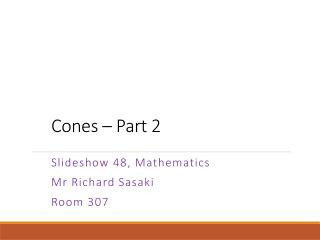DownloadDownload PresentationCones – Part 2

# Cones – Part 2

Download Presentation## Cones – Part 2

- - - - - - - - - - - - - - - - - - - - - - - - - - - E N D - - - - - - - - - - - - - - - - - - - - - - - - - - -
##### Presentation Transcript

1. Cones – Part 2 Slideshow 48, Mathematics Mr Richard Sasaki Room 307

2. Objectives • Review the properties of a cone and formulae we have learned about their properties • Be able to make calculations about attributes of cones regarding both their bases and lateral surfaces

4. The Net for a Cone Let’s have a quick review. Lateral Surface Radius / Radii Base Central angle(s) (Area) Sector(s) (Area) Arc Length

5. The Net for a Cone The net for a cone is formed by two things. A and a . circle sector If we have information regarding both…what is one common piece of information with the same name? The radius!! Oh no! It’s crazy and confusing so we will only consider the cone as a shape, not as its net.

6. The Cone When the cone is produced, what is the name of the radius of the sector? The slant height! Let’s review the properties of a cone. Two pieces of information (not labelled) are identical. What are they? Slant height - The circumference of the base and arc length of the sector!

7. Finding Things… Have a look at this cone. We’re given two pieces of information. The radius of the base. The slant height. How could we calculate the circumference at the base? the lateral surface’s arc length This is also .

8. Finding Things… How about the angle at the apex formed by the lateral surface? Do you remember the formula we need? We need the formula to find the circumference of the base.

9. Answers (Section 1) C = cm C = cm +=

10. Shortcuts… We know and and these are fine to use to calculate the surface area when we are given and . But it’s a long process, right? Let’s check our process with Q3. Surface Area = A + S

11. Shortcuts… Surface Area = A + S Surface Area (cone) =

12. Example Knowing shortcuts makes things easier! But of course you have to remember them. Example A cone has radius 5cm and slant height 8cm. Calculate its surface area.

13. Answers - Easy S.A = cm2 S.A = cm2 S.A = cm2 S.A = cm2 S.A = cm2 Tall and thin. S.A = cm2

14. Answers - Hard S.A = cm2 Because both start at the same place and reach the middle but the slant height must reach a higher point too. S.A = = = S = S.A = No. (Reasoning is the same as Question 2.) C S.A = S.A =